Next: Spin Magnetic Resonance Up: Time-Dependent Perturbation Theory Previous: Preliminary Analysis

# Two-State System

Consider a system in which the time-independent Hamiltonian possesses two eigenstates, denoted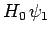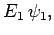(1031)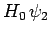(1032)

Suppose, for the sake of simplicity, that the diagonal elements of the interaction Hamiltonian,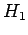, are zero: i.e.,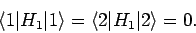(1033)

The off-diagonal elements are assumed to oscillate sinusoidally at some frequency: i.e.,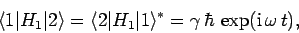(1034)

where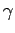andare real. Note that it is only the off-diagonal matrix elements which give rise to the effect which we are interested in--namely, transitions between states 1 and 2.

For a two-state system, Eq. (1028) reduces to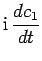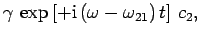(1035)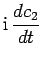(1036)

where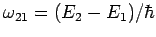. The above two equations can be combined to give a second-order differential equation for the time-variation of the amplitude: i.e.,(1037)

Once we have solved for, we can use Eq. (1036) to obtain the amplitude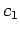. Let us search for a solution in which the system is certain to be in state 1 (and, thus, has no chance of being in state 2) at time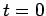. Thus, our initial conditions are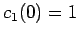and. It is easily demonstrated that the appropriate solutions to (1037) and (1036) are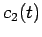(1038)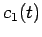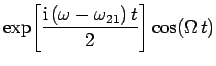(1039)

where(1040)

Now, the probability of finding the system in state 1 at timeis simply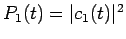. Likewise, the probability of finding the system in state 2 at timeis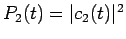. It follows that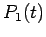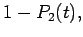(1041)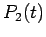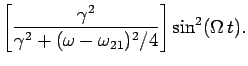(1042)

This result is known as Rabi's formula.

Equation (1042) exhibits all the features of a classic resonance. At resonance, when the oscillation frequency of the perturbation,, matches the frequency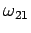, we find that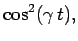(1043)(1044)

According to the above result, the system starts off in state 1 at. After a time interval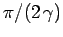it is certain to be in state 2. After a further time intervalit is certain to be in state 1 again, and so on. Thus, the system periodically flip-flops between states 1 and 2 under the influence of the time-dependent perturbation. This implies that the system alternatively absorbs and emits energy from the source of the perturbation.

The absorption-emission cycle also takes place away from the resonance, when. However, the amplitude of the oscillation in the coefficientis reduced. This means that the maximum value ofis no longer unity, nor is the minimum ofzero. In fact, if we plot the maximum value ofas a function of the applied frequency,, we obtain a resonance curve whose maximum (unity) lies at the resonance, and whose full-width half-maximum (in frequency) is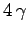. Thus, if the applied frequency differs from the resonant frequency by substantially more than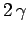then the probability of the system jumping from state 1 to state 2 is always very small. In other words, the time-dependent perturbation is only effective at causing transitions between states 1 and 2 if its frequency of oscillation lies in the approximate range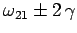. Clearly, the weaker the perturbation (i.e., the smallerbecomes), the narrower the resonance.Next: Spin Magnetic Resonance Up: Time-Dependent Perturbation Theory Previous: Preliminary Analysis
Richard Fitzpatrick 2010-07-20